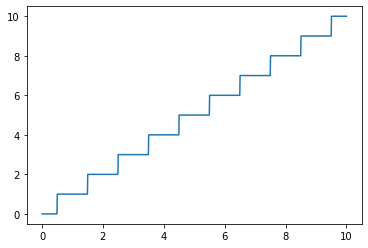# Model parameters doesn't change when training

Hey guys,

I’m working with a simple model which has to train 1 value only, named `self.my_weights` in my below code. The only new thing in my experiment is that I have to round that value when forwarding the input.

In my observation, that value doesn’t change anything when training. Assume the loss function is working. Does my “rounding values” action make that tensor un-trainable? Do I have any wrong implementations? Pls help!

This is an example of my network:

``````class MyModel(nn.Module):

def __init__(self):
super(MyModel, self).__init__()
self.backbone = MobileNetv3(pretrain=True)  #This self.backbone's weights are frozen when training
self.backbone.classifier = nn.Sequential()

def forward(self, input):
feature = self.backbone(input)
repeat_weights = torch.round(self.features_weights).to(torch.int64)

final_features = feature.repeat(1, repeat_weights))

final_features = F.normalize(final_features, dim=1)
return final_features
``````

Yes, for two reasons:
Rounding a value will create a step function. While the output will still be attached to the computation graph, the gradient will be zero almost everywhere:

``````x = torch.linspace(0, 10, 1000)
y = torch.round(x)

plt.plot(x.numpy(), y.numpy())
``````

Output:Afterwards you are transforming the output to an integer type (`torch.int64` in this case), which will explicitly detach the result from the computation graph since only floating point tensors are differentiable.

1 Like

Thanks @ptrblck for your magnificent explanation!

I’m working around to find a method to make it works. My purpose is to train a repeat value as the parameter for torch.repeat() function.

Unfortunately, `torch.repeat()` requires an `int` number for its parameter, and the `self.my_weights` in my code has to be `float` tensor to be trainable.

It seems to be unrealizable here!